# Smoothing a Column of Data## Overview

#### Synopsis:

Just as we smooth images to enhance details, we can also smooth columns or arrays. Also, just like when working with smoothed images, we must be careful that any results are not artifacts from the smoothing process.

Most users will probably use ds9 to smooth images or if ambitious use the csmooth tool. The tools to smooth column or 1D data have existed since the first CIAO release however they have not been highlighted in threads or other documents and therefore may be unknow even to veteran CIAO users.

In this thread we will introduce the aconvolve tool which performs traditional linear smoothing (Gaussian) as well as the newer dmtabfilt tool which can apply various non- linear filters (e.g. quantile calculation).

#### Purpose:

Users may follow this thread if they want to smooth a column in a file (or 1D array of values).

Last Update: 15 Feb 2021 - Review for CIAO 4.14. Updated for Repro-5.

## Getting Started

`unix% download_chandra_obsid 14382 mtl`

To make the plotting less cluttered we subtract off the start time of the observation from the TIME values in the mission time line.

```unix% dmtcalc acisf14382_000N002_mtl1.fits"[cols time,fp_temp]" \
fptemp.fits exp="time=time-tstart" ```

We will use fptemp.fits in the rest of this thread.

The fp_temp column is the ACIS focal plane temperature. Various calibrations depend on the FP_TEMP value making it an interesting value to study.

## Plotting the raw data

We begin by simply plotting the FP_TEMP values vs TIME to look for any interesting features.

```unix% python
>>> import matplotlib.pylab as plt
>>>
>>> tt = tab.get_column("time").values
>>> temp = tab.get_column("fp_temp").values
>>>
>>> plt.plot(tt,temp,linestyle="", marker="o", markersize=2)
>>> plt.xlabel("Time [sec from TSTART]" )
>>> plt.ylabel("Focal Plane Temperature [K]")
>>> plt.title("Observation ID : 14382" )
>>> m = max(temp)
>>> n = min(temp)
>>> plt.fill([4000,7000,7000,4000], [n,n,m,m], color="grey", alpha=0.5 )
```

The plots in Figure 1 suggest that there might be some quantization or digitization effects where the temperature is osciallating between two different values. However, given the quantization noise and scatter in the plots, it is difficult to discern if a trend exists.

### Figure 1: Data from the Mission Time Line file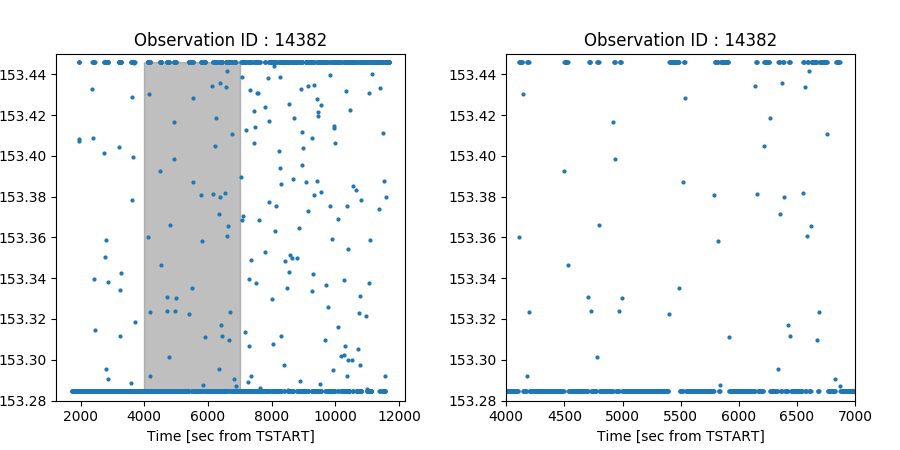### Figure 1: Data from the Mission Time Line file

The focal plane temperature vs. time for ObsID 14382. (Left) shows the full range of data and (Right) shows the same data zoomed in on the highlighted time range -- lines are added to help guide they eye from point to point. The FP_TEMP values vary between two levels with some scatter inbetween. The scatter is due to the interpolation of the raw engineering data onto the times used in the mission time line; the data really is showing two discrete temperature values. The density of the points at the beginning of the observation compared to the end suggests there might be a trend, but it is difficult to see given the noise and quanzitions effects.

If we smooth the data it will do two things:

1. Reduce the scatter in the plot.
2. Essentially interpolate between the two discrete values.

## Linear Smoothing

### Box

The most common type of smoothing for 1D datasets is a "running mean", "moving average" or "window mean" where the average value within some number of adjacent rows is computed and replaces the current value. The only parameter is how large the window should be.

In terms of smoothing or convolution, this is achieved by smoothing the data with a box (or boxcar) shaped kernel whose values are all 1/N, where N is the length of the smoothing window. We often choose N to be an odd number so that equal number of rows before and after the current value are used.

The box is one of the library options available in aconvolve.

The syntax for the box kernelspec is: lib:box(dimension,amplitude,length_i,...)

• lib : designates a built in library function, other options are file and text
• box : the shape of the kernel in the library. There are several built in kernels
• dimension : "1" in our case since we are smoothing a column. If smoothing 2D images, use 2
• amplitude : "1" the maximum pixel values, before it is renormalized. With the aconvolve normkernel=area (default) this value is not important (should simply be greater than 0)
• length_i : the length of the box. For images, 2 lenghts are supplied.

We can now smooth the FP_TEMP values in the mission time line using the following aconvolve command:

```unix% pset aconvolve infile="fptemp.fits[cols time,fp_temp]"
unix% pset aconvolve outfile=mean_fptemp.fits
unix% pset aconvolve kernelspec="lib:box(1,1,51)"
unix% pset aconvolve meth=slide edge=nearest norm=area clob+
unix% aconvolve mode=h
```

We explicitly gave the column names because aconvolve will smooth the 2nd column in the table (commonly the Y-axis) and will only run a cursory data check (equally spaced values) on the 1st column (X-axis). Using similar plotting commands to above we get the plot shown in Figure 2

### Figure 2: Mean Smoothed Data from the Mission Time Line file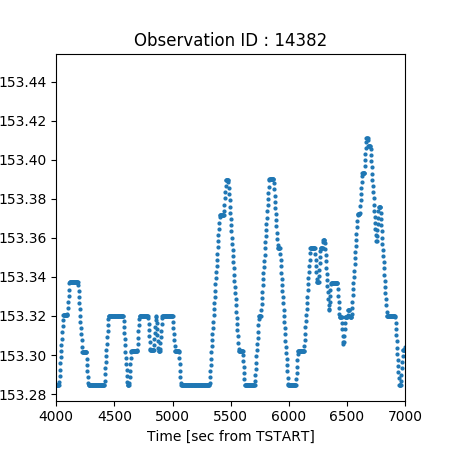### Figure 2: Mean Smoothed Data from the Mission Time Line file

Using aconvolve we compute the mean of the FP_TEMP values in a sliding window that is 51 rows long. We can start to see a clear trend: the FP_TEMP is increasing with TIME, but there is still a lot of quantization noise.

After the boxcar smoothing, there is a clear trend emerges: the FP_TEMP is increasing with time. There are however still some quantization effects and a periodic pattern may be present.

To further investigate these, we can choose a smoothing kernel that weights data points further away from the center less than values closer to the center. The most common such kernel is the Gaussian.

### Gaussian

Gaussian smoothing in 2D is very common; less so in 1D though the same underlying reasoning remains. If the signal you are looking for has some sort of center-peaked feature, convolving with something with a similar shape will accentuate that feature (remember that convolution and correlation are the same for symmetrical kernels). Because the central limit theorem ensure that Gaussian smoothing arises in nature, it is common to use a Gaussian for smoothing.

aconvolve also has a lib:gaus built in smoothing kernel. The parameters are slightly different than for the box. It takes the form: lib:gaus(dimension,n_sigma,amplitude,sigma_i)

• lib: same as for box (above)
• gaus: Gaussian function: N*e^(-x/σ)
• dimension: same as box (above)
• n_sigma: truncate the Gaussian at a radius > this number of σ.
• amplitude: same as above for box
• sigma_i: The σ (not FWHM) of the Gaussian along each dimension.

First we will try a σ of 21 rows.

```unix% aconvolve fptemp.fits"[cols time,fp_temp]" gaus21_fptemp.fits \
kernelspec="lib:gaus(1,5,1,21)" meth=slide edge=nearest clob+```

The resulting plot shown in Figure 3 (left), shows the same overall increasing trend. It also shows what appears to be even more evidence of periodicity. However, one should be careful about reading too much into the data given how it has been processed. In particular if the periodicity matches the size of the convoluiton kernel, either we got very lucky and tuned our Gaussian to act as a matched filter or the result is simply due to how the data we sampled. In this case, one should be very suspicious given the amount of quantization in the original data and the amplitude of the apparent periodicity.

We can smooth with a different sized kernel and if the periodicity remains we may have more confidence in its presence. We repeat with the σ of 51 rows:

```unix% aconvolve fptemp.fits"[cols time,fp_temp]" gaus51_fptemp.fits \
kernelspec="lib:gaus(1,5,1,51)" meth=slide edge=nearest clob+```

From Figure 3 (right), one may argue that the peridoicity is still present after heavily smoothing. Still the amplitude is very small compared to the quantization levels so one should look for more evidence.

### Figure 3: Gaussian Smoothed Data from the Mission Time Line file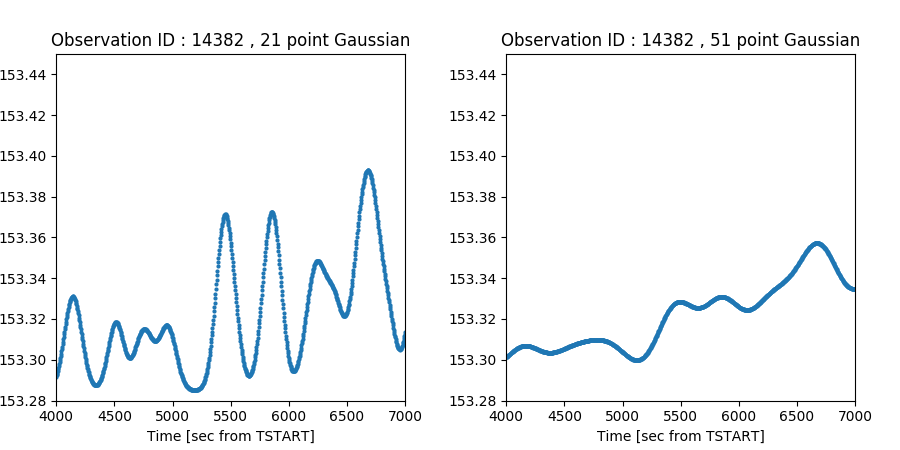### Figure 3: Gaussian Smoothed Data from the Mission Time Line file

Applies a Gaussian weighting to data points. Points further away from the center are weighted less than current point. (Left) is using a 21 σ row Gaussian kernel. (Right) is using a 51 σ row Gaussian kernel.

As mentioned before, the scatter between the two quanzated FP_TEMP values is due to the irregularlly spaced in time FP_TEMP values being interpolated onto a regularly spaced grid in the mission time line.

Could the periodicity be coming from some harmonic interaction between the smoothing scale and the interpolation grid spacing?

## Non Linear filtering

Both the box and Gaussian smoothing are linear filters: they are linear combination of values in the column. They just weight the data points differently.

However, all linear filters have problems representing the true underlying signal when (a) the length of the filter is short and (b) when there are large noise spikes.

To deal with these kinds of data there are many non-linear techniques that can be used. Non-linear simply means that the output is not a linear combination of the input. Functions such as min and max are non-linear: the output is not a linear combination of the inputs.

### Olympic / Fancy Mean

One of the most simple kinds of non-linear filters is the Olympic style mean. To suppress the effects of outliers, the min and max values are removed from the sample and the mean is taken from the remaining values. This approach has been used for years by the Olympic judges to suppress national biases.

While this kind of smoothing is a linear filter in the same sense as the mean is a linear filter, it is non-linear in that min and max rows which are excluded are not the same for every data point.

We can use the dmtabfilt tool to apply an olympic smoothing to the FP_TEMP data like this

```unix% pset dmtabfilt dmtabfilt infile=fptemp.fits
unix% pset dmtabfilt out=temp_out
unix% pset dmtabfilt col=fp_temp
unix% pset dmtabfilt function=olympic
unix% dmtabfilt mode=h
unix% dmpaste fptemp.fits temp_out fp_temp_olympic101 clob+```

The input to dmtabfilt is the column name, the filtering function (in our case olympic), and a mask that is a series of 1's and 0's that selects which rows are used (the mask is always centered at the current row so should have an odd number of values).

The output from dmtabfilt is a table with a single column whose name is a concatenation of the original column and the filter applied; so in this example fp_temp_olympic. To make plotting and analysis easier, we use dmpaste to paste this column back onto the original file.

In this example we will use a mask with one hundred and one values of "1", which means the olympic mean is taken by examining the 50 rows before, 50 rows after, and the current row. The ")" syntax is a parameter redirect that retrieves the parameter named 101 from the dmtabfilt parameter file.

```unix% plist dmtabfilt
Parameters for /home/user/cxcds_param4/dmtabfilt.par

infile = fptemp.fits      Input table file name
colname = fp_temp          Column to smooth
outfile = temp_out         Output file name
function = olympic          Filter function
(verbose = 0)               Tool verbosity
(clobber = yes)             Clobber existing output?
(mode = ql)
(3 = 111)
(5 = 11111)
(7 = 1111111)
(9 = 111111111)
(11 = 11111111111)
(21 = 111111111111111111111)
(101 = 11111111111111111111111111111111111111111111111111111111111111111111111111111111111111111111111111111) ```

The results of the olympic filtering can be compared to simple mean in Figure 4. (Left) is the olympic mean which has the highest and lowest values excluded from the mean compared to the (Right) simple mean which shows stronger spikes.

### Figure 4: Olympic smoothing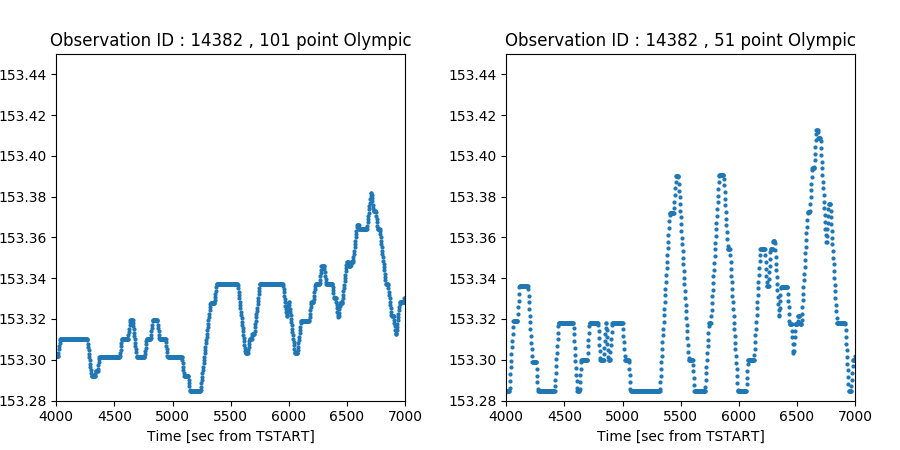### Figure 4: Olympic smoothing

(Left) The 101 point Olympic mean filter applied to the FP_TEMP data compared to (Right) the simple mean (same as Figure 2).

### Quantiles

We learn early in statistics that under the circumstances mentioned above that the median value may be a better approximation to the true value as it naturally suppresses outliers. The median is of course just a special case of what is commonly known as quantile analysis.

In quantile analysis we order our data and pick the value that represents some percentage of the data.

For the median of a column data we numerically sort the values and return the data point at the 50% point. That is 50% of the data in the array have a value less than the median value. This will tend to suppress spikes that only have a few (say larger) values that do not affect the median as dramatically as it would the mean.

We can use the dmtabfilt tool to do a "running median" similar to the "running mean" illustrated above.

```unix% pset dmtabfilt infile=fptemp.fits
unix% pset dmtabfilt outfile=temp_out
unix% pset dmtabfilt colname=fp_temp
unix% pset dmtabfilt function=median
unix% dmtabfilt mode=h
unix% dmpaste fptemp.fits temp_out fp_temp_median101 clob+```

The result of the 101 point median filter can be seen in Figure 5 (left). dmtabfilt has several other common quantile levels available: 25%, 33%, 67%, and 75%. We can repeat this using the 75% quantile filter function, q75.

```unix% dmtabfilt in=fptemp.fits out=- col=fp_temp function=q75 mask=")101" | \
dmpaste fptemp.fits - fp_temp_q75101 clob+```

In the above example we make use of the special "-" outfile name which lets use pipe the output from dmtabfilt to dmpaste without having to write an intermediate file. The results of the q75 filter are in Figure 5 (right).

### Figure 5: Quantile based smoothing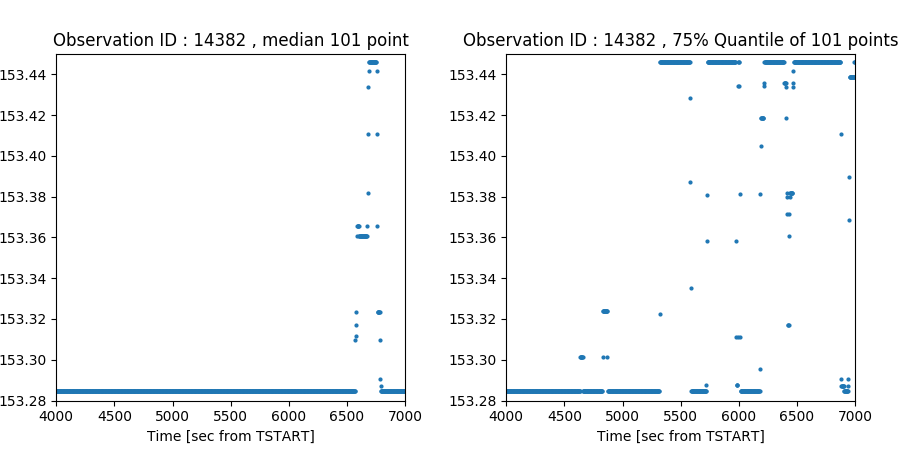### Figure 5: Quantile based smoothing

(Left) The 101 point median filter of the FP_TEMP values. The scatter between the two quantized values has been signficantly reduced. (Right) The 101 point 75% quantile value, that is 75% of the values in a 101 row window centered on the current row are below the plotted value.

### Extreme

For these specific data, we know that values scattered between the two limits are an artifact of how they were interpolated onto the time grid used in the mission time line file. We might want to try to recover the original quantized values.

We can use the extreme filter with a short mask (ie small window) to recover the original quantization levels. The extreme filter computes the mean of the values in the mask. If the mean is closer to the minimum value in the mask, it sets the output row to the min. If the mean is closer to the maximum, then the output row is set to the maximum.

```unix% dmtabfilt in=fptemp.fits out=- col=fp_temp function=extreme mask="111" | \
dmpaste fptemp.fits - fp_temp_extreme clob+```

The results of are shown in Figure 6 (left). We used a short mask="111", only 3 rows (the current, previous, and next rows) so only the scatter between the two original FP_TEMP values have been suppressed.

We can further filter these values say with the median filter as above.

```unix% dmtabfilt in=fptemp.fits out=- col=fp_temp function=extreme mask="111" | \
dmtabfilt in=- out=- col=fp_temp_extreme function=median mask=")101" | \
dmpaste fptemp.fits - fp_temp_extreme_median clob+```

In this example we can see the convience of using the "-" to pipe outputs from tool to tool without having to write intermediate files. The result of this two pass smoothing are show in Figure 6 (right).

### Figure 6: Extreme smoothing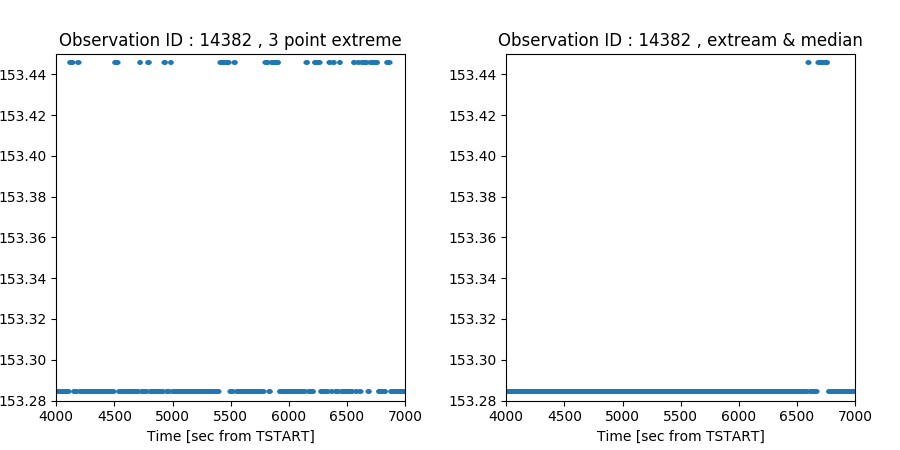### Figure 6: Extreme smoothing

(Left)The FP_TEMP data smoothed with the extreme non-linear filter. The min or max value closest to the mean is used. (Right) The result of taking (left) and further smoothing with a median filter. The first filter removes the scatter between the two quantized values and then the second removes most of the short-time scale fluctuations.

### Summary

In this thread we have demonstrated some of the smoothing techniques for tabular or array available in CIAO. When such smoothing should be applied and how the results are to be interpreted depend on the data being smoothed and science being investigated.

In general smoothed data are heavily processed. While smoothing may suppress some data artifacts it may introduce others. Users should use the smoothed data to guide analysis and not necessarily to be used as the sole basis for scientific discovery.

## History

 25 Feb 2013 Initial version. 11 Dec 2013 Review for CIAO 4.6; minor edits. 23 Dec 2014 Review for CIAO 4.7; no changes. 01 Feb 2016 Updated ds9 links. 08 Apr 2019 Update plots with matplotlib. 15 Feb 2021 Review for CIAO 4.14. Updated for Repro-5.